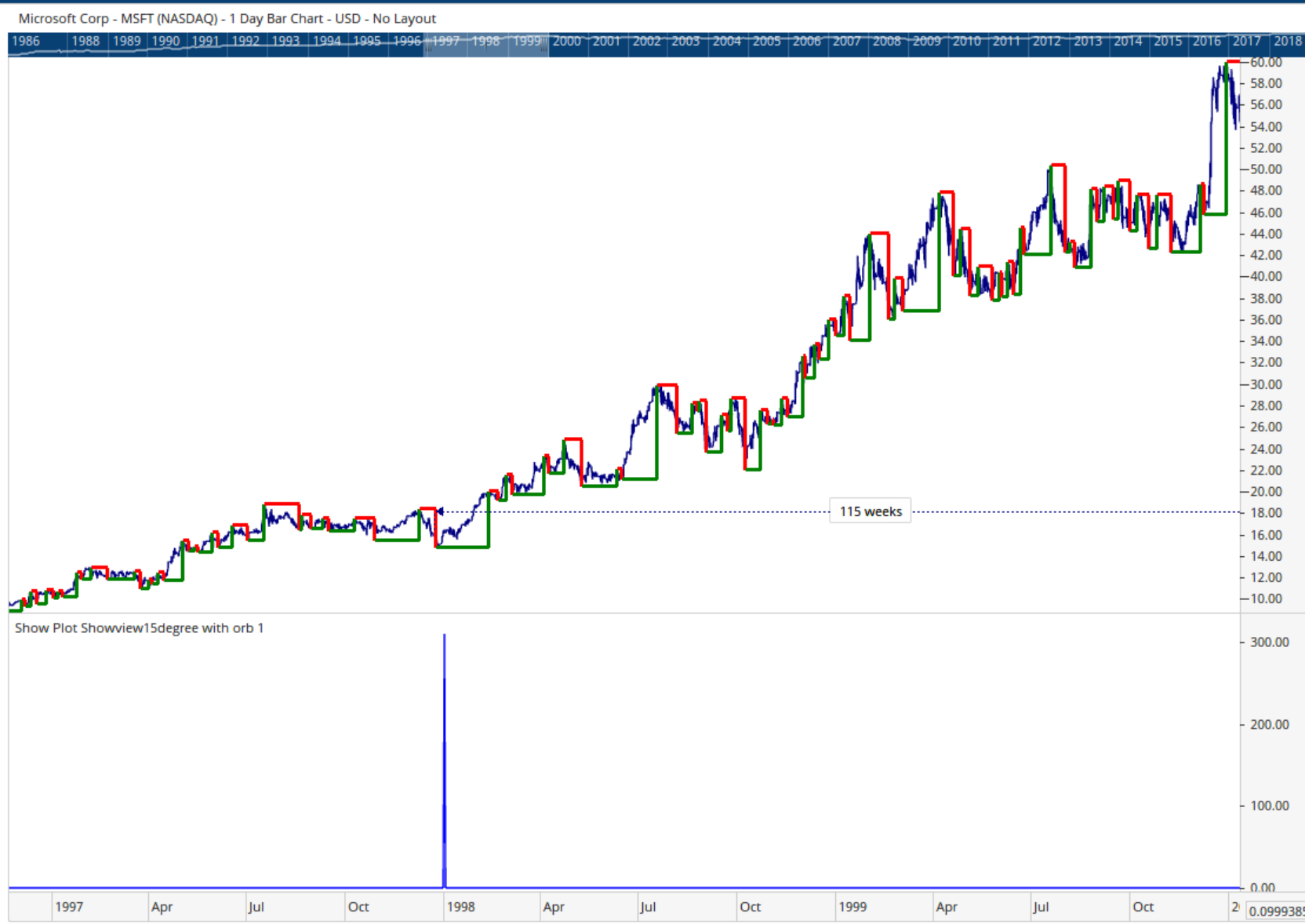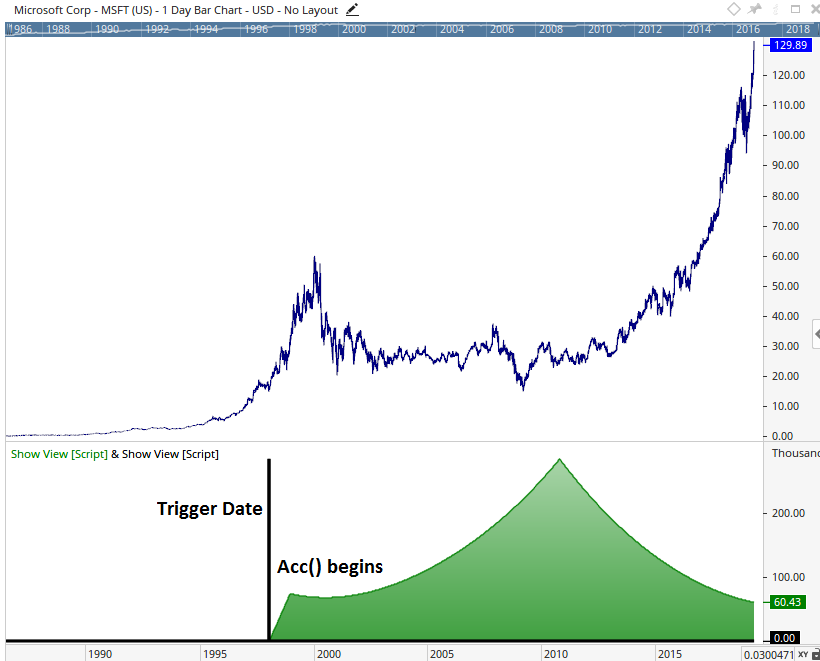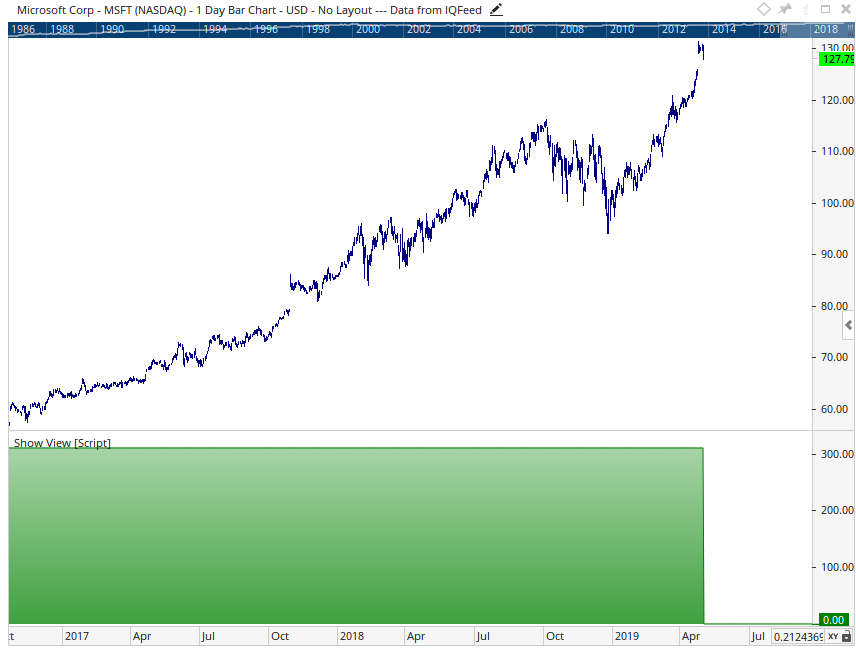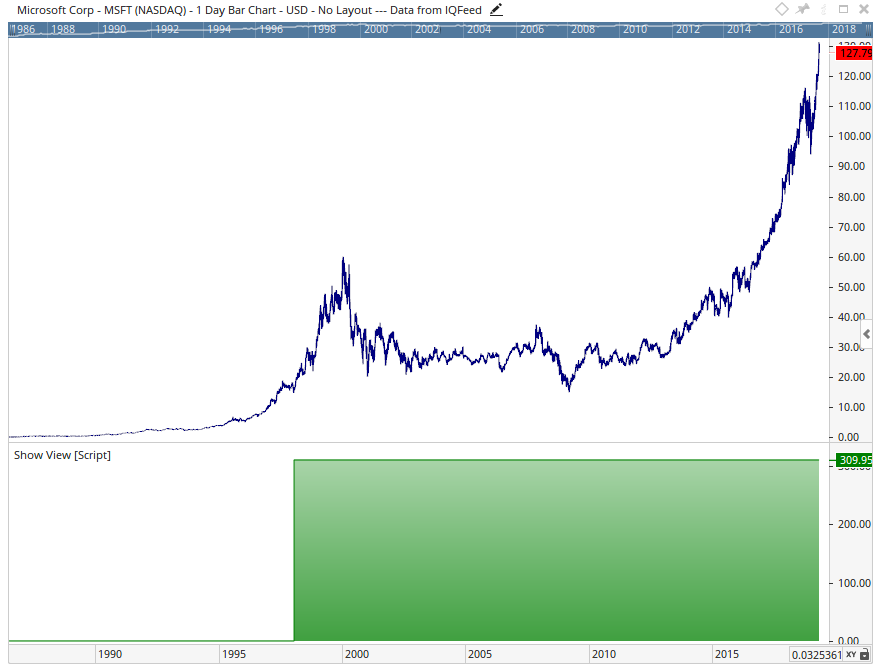Select Page

# Accumulation ACC() does not seem to work

Optuma Forums Optuma Scripting Accumulation ACC() does not seem to work

Viewing 6 posts - 1 through 6 (of 6 total)
• Author
Posts
• #52873

Hi there,

I am trying to accumulate a determined value, but for some reason it does not seem to accumulate the value see graph and code below, Have I entered in the code correctly? I have also tried (FIRST(Nonzero(f2)); But the value still drops back to zero after the start date.

star_date = 35795;

p1 = PVAL(PLANET=[Jupiter], VALUE=Longitude, GEO=HelioCentric, GMT=-5:00) ;

p2 = PVAL(PLANET=[Saturn], VALUE=Longitude, GEO=HelioCentric, GMT=-5:00);

v1 = p1-p2;

v2 = if( bardate() == star_date, v1,0);

f2=v2;

ACC(f2);#52875

Hi Mario,

If you are wanting to accumulate the value of V1 from the specified Bar Date in the script, rather than using a == (which means a value is returned on 1 bar only) adjust it to >= so any date on or after the trigger is included in the accumulation.

The updated result looks like this:#52931

Great, thank you Matthew 🙂

#52941

Hi Matthew,

What I was trying to do, was get the value on that specific date, and then use it as a reference on every bar from that point forward, so if the value was 250, I wanted to assing f2 to 250, and then be able to reference back to the 250 on each bar.  What is happening in the code, is that f2 is equal to 250 on the first bar, but then changes to 0 the next bar, as the start date no longer matches.  In order to carry the 250 to each subsequent bar, i was trying to accumulate the f2 , hoping that it would take the initial value of 250 and add 0 to this on each bar, keeping 250 as the constant result.  In the above, what happens is that the 250 is fluctuating with each new bar, as the degrees change, which is then not providing me with the reference back to the 250.  I have tried to use FIRST(Non zero, f2) but still does not seem to carry this value of 250 across to the future bars.  Not sure if there is a logical error here, but logic seems correct, just cant seem to get it to work in the code.

Thanks a mil

Mario

#52943

Hi,

First() and Nonzero() should be used to achieve the result you’re after, i think the problem is you’re trying to wrap it account the ACC() function which isn’t needed in this case.If you need the value to be zero until the selected bar date, the script would need to use the following:#52979

Perfect, thanks Matthew, I will try that out.

Viewing 6 posts - 1 through 6 (of 6 total)
• You must be logged in to reply to this topic.